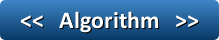## Pages

### AlgorithmWhat is an algorithm?

Algorithm is a procedure or step by step process for solving a problem.
• Algorithms can be expressed using different kinds of notations, including pseudocode, flowcharts, programming languages and natural languages.
• Computer algorithm is an instance of logic written in computer software by the programmers.

Let’s learn some of the algorithm. Sample problem definition and algorithm is given below:

1. Problem Definition: Write an algorithm to add given two numbers.

Step 1: Start.
Step 2: Read two numbers A and B.
Step 3: Total = A+B.
Step 4: Display Total.
Step 5: Stop.

Click here to learn how to convert this algorithm in to C program.

2. Problem Definition: Write an algorithm for Subtracting two Numbers.

Step 1: Start.
Step 2: Read two numbers A and B.
Step 3: Answer = A - B.
Step 4: Display SUB.
Step 5: Stop.
3. Problem Definition: Write an algorithm to find factorial of a given number.

Step 1: Start
Step 2: Declare variables: N, fact,  i.
Step 3: Initialize variables
fact = 1
i = 1
Step 4: Read value of N from user.
Step 5: Repeat following steps until i = N
fact =  fact * i
i = i + 1
Step 6: Print fact variable.
Step 7: Stop

4. Problem Definition: Write an algorithm to multiply given two numbers.

Step 1: Start.
Step 2: Read two numbers A and B.
Step 3: Answer = A * B.
Step 5: Stop.

5. Problem Definition: Write an algorithm for dividing given two numbers.

Step 1: Start.
Step 2: Read two numbers A and B.
Step 3: Answer = A / B.